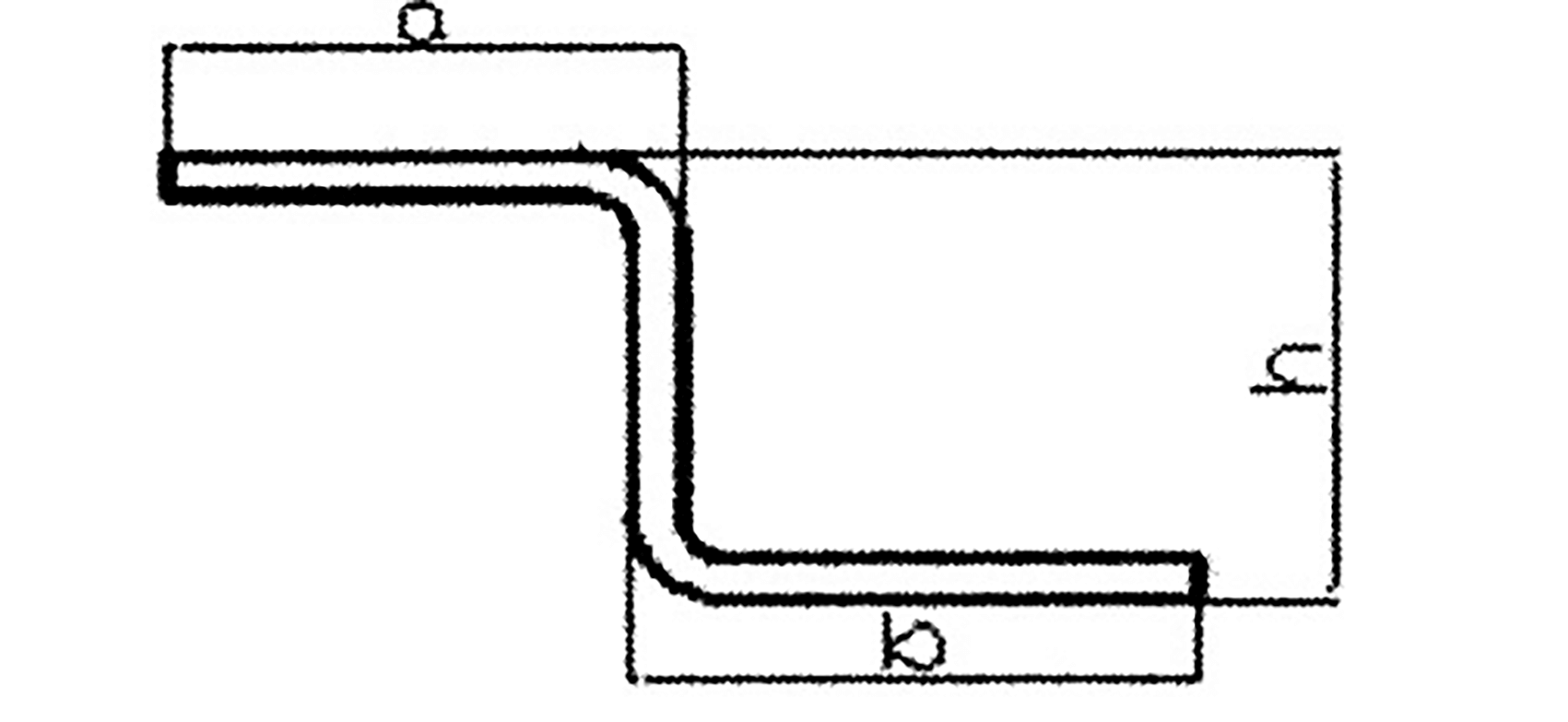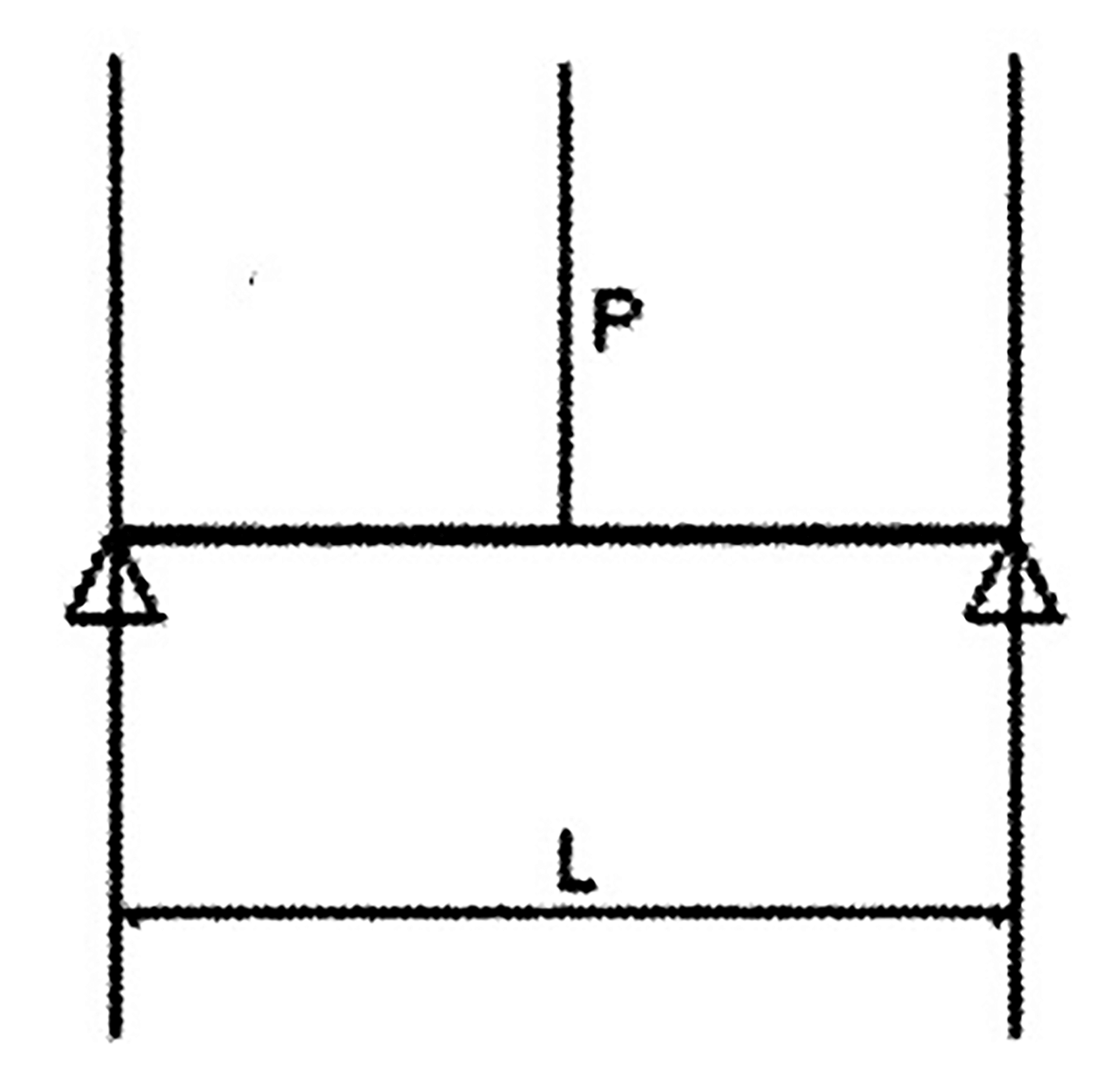# Z-shaped Bending and the Forming Die (Structure Analysis)

## 1. Overview

The Z-shaped bending workpiece shown in Figure 1 is a part frequently encountered in the production, where the size of h is limited by the lower die, the existing lower die can bend the minimum size of h as shown in Table 1.

Table 1 Minimum size bending

 Plate thickness 1 1.5 2 2.5 3 Minimum size 7 9.5 14 16 18

In actual production, if there are a large number of Z-shaped bending dies that are smaller than the above size, it would need to design a composite bending die to achieve primary molding with multi-purpose, folding Z-shaped bending dies of different sizes on different sheet materials.Fig. 1 Z-shaped bending die

## 2. The process of bending deformationFig. 2 Action force diagram

As shown in Figure 2: when sheet metal is bent, it bears bending moment, shear force and local pressure, but the main effect of bending deformation is bending moment.

Under the action of the external force, the corresponding deformation will inevitably occur.

At the same time, the internal force resisting deformation will appear in the sheet metal.

This internal force is balanced with the external force (the internal force per unit area of the object is called stress).

The greater the external force, the greater the stress and the greater the deformation.

When the outer stress of the material is less than the elastic limit of the material, the sheet metal is in the state of elastic deformation.

According to Hooke’s law, the distance between the deformation (outer layer lengthening, inner layer shortening) and the central layer changes linearly, so the stress on the section also changes linearly (as shown in Fig. 2),

If the external force is removed, the sheet metal can return to its original shape.

If the external force continues to increase, the deformation degree of the bending part will continue to increase until the stress caused by the external force is equal to the yield limit of the material, and the plastic deformation of the outer material occurs.

With the increase of external force, the plastic deformation develops from the surface to the center.

At this point, if the external force is removed, the elastic deformation in the sheet disappears immediately.

However, the plastic deformation is preserved, resulting in permanent bending deformation.

When the stress caused by the external force is greater than the strength limit of the material, the sheet metal will develop from plastic deformation to fracture.

The bending of sheet metal under inner compression also produces plastic deformation, but this kind of plastic deformation increases the stress surface without causing damage, which is usually ignored.

## 3. Calculation method of bending

Now we carefully observe the plastic bending deformation.

Under the action of bending moment, there are three equal lines on the plate section: ab= a1b1 = a2b2.

After bending, the inner layer shortens and the outer layer elongates, that is, ab < a1b1 < a2b2.

Therefore, when bending, the inner material is compressed and shortened, while the outer material is stretched and elongated.

Then, between tension and compression, there must be a layer of material that is neither stretched nor compressed.

Its length does not change, neither elongate nor shorten, and this layer is called neutral layer.

The method of calculating the bending part is to divide the bending part into several basic geometric elements of straight line segment and arc segment.

The length of each element is calculated respectively, and then the total length of each element is calculated, which is the unfolded length of the bending part.

The Z-shaped bending part shown in Figure 1 can be divided into five units according to figure 2.

1, 3 and 5 are straight line segments, and 2 and 4 are arc segments.

It can be seen from the above discussion that the fiber layer with constant length in the middle before and after bending is called neutral layer.

When we calculate the expansion length of the arc segment, it actually calculates the neutral layer length of the arc segment.

The position x of the neutral layer from the inner side of the arc (as shown in Figure 3) is generally determined by the r/t ratio.

x = kt

Where:

• t — Material thickness
• k — Neutral layer position coefficient (or neutral layer coefficient)
• k = R – r/t
• R = r + kt

Where:

• R — The distance from the center of radius r to the bending neutral lineFig. 3

The value of k varies with the ratio of inner radius to sheet thickness, shown as Table 2:

Table 2

 r/t 0.25 0.5 1 2 3 4 k 0.26 0.33 0.35 0.375 0.4 0.415

In the production practice, it is most common to fold the steel plate into a right angle shape.

The calculation of the arc length of 90 ° bending for different r and t can be obtained by looking up the table in practical application.

This article focuses on 90 ° right angle bending, but it is not convenient to calculate the straight line and arc separately for the parts with 90° sheet metal bending.

Instead, it is marked as shown in Fig. 1.

When we calculate the unfolding material, we directly use the marked dimensions to obtain a simple calculation method.

According to figure 1, the developed material length is calculated:

L = a＋b＋h – 2x

Where:

• x — Common bending coefficient

## 4. Structure and working process of Z-shaped bending compound die

Table 3 Common bending coefficient x

 Plate thickness t Inner bending radius r 1.0 1.5 2.0 2.5 0.5 1.0 1.2 1.4 1.6 1 1.9 2.1 2.3 2.5 1.5 2.5 2.7 2.9 3.1 2.0 3.4 3.6 3.8 4.0 2.5 4.0 4.2 4.4 4.6 3 4.9 5.1 5.3 5.4

This simple set die is different from the regular die.

The design requires simple, fast, easy to process and easy to form.

It is slightly inferior to the regular die in accuracy, but it is applied to the processing of the products quickly and accurately.

The forming diagram of the compound die is shown in Table 3.

Processing principle:

The thickness of the gasket is adjusted to achieve the required width of the V groove of the upper and lower die, and to form one-time Z fold processing under pressure.

Die structure: Z-shaped bending die consists of upper die, lower die, gasket and angle prism steel.

Gasket thickness: The thickness of the spacer is 0.5mm thick steel, which is superimposed to achieve the required thickness.

Prism steel: A rectangular steel in the die, its four angles are chamfered into four sides of 0.5 mm, 1.0 mm, 2.0 mm and 4.0 mm, as shown in Table 3.

Using a special simple die, the desired width of the upper and lower die V-groove is achieved by adjusting the size of the angular prism steel and the thickness of the gasket, being pressurized to form the Z-fold processing at once.

It is selected because of the possibility of increasing the V-slot and reducing the crease, so different plate thickness needs to choose different prongs, as shown in Table 4:

Table 4

 Plate thickness t<0.8 0.8

Debugging method of Z-shaped bending die:

1) Both folds of the straight-edge Z-fold are 90°.

The distance between the two tool tips is: 1.414/2×h;

2) If the workpiece crease is too deep, then a needs to choose a large angle.

b: pad iron; c: increase angle R;

3) If the height is reached, but the angle is greater than 90°, then a: die eccentricity.

b: Increase the thickness of the shim;

4) If the two sides of the Z-fold are not parallel, it can be achieved by increasing or decreasing the thickness of the shim.

If the upper fold is greater than 90°, the thickness of the lower die shim needs to be increased; and if the lower fold is greater than 90°, the thickness of the upper die shim needs to be increased.

Z-bend expansion calculation method:

When h > normal bending size, it should unfold by two folds.

L=a + b + h – 2x

Where:

• l — Length of unfolded material

When h < the normal bending size, it is expanded by one-step forming.

L = a + B + h – 1.5x

Where:

• x — Common bending coefficient

One of the empirical formulas in practice is to subtract 1.5x from the overall dimension of one molding.